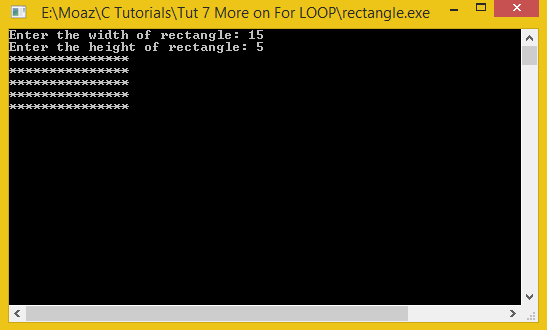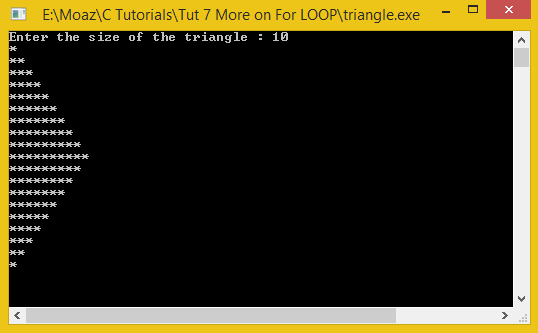# Nested For LOOPS

In this part you will learn:

1. For LOOP
2. Nested For LOOP
3. More on conditional statements
4. C syntax
5. Showing output

In this tutorial I will teach you how to make different shapes using For Loop and * . Making different shapes with * may sound something like very unimportant but trust me making these shapes provide a very good understanding of the loop.

What is a NESTED LOOP ?
Sometimes we need two loops to execute a statement. For example if we want to solve a 3 x 3 matrix then we will need two loops one for rows and other one for columns. So we use a loop within a loop for every iteration of the parent loop the inner loops runs completely.
Eg: for(int i=1;i For(int j =1;j Printf(“*”);
}
It will print 100 astericks because for each int i , int j runs 10 times.
Making a rectangle with astericks using nested FOR LOOP:

Basic Step:
Open Dev C++ then File > new > source file and start writing the code below.

`#include<stdio.h>#include<conio.h>`

These are the header files that we need for coding. The main header is stdio.h which provides us with most of the functions for coding in C and the second header files is just for using a function which can pause the screen until the user hits any key.

`int main(){		int width,height; // dimensions	int w,l;`

For making a rectangle we need to take input two variable i.e width and height. So we declare them first and we also declare the loop counters.

`	printf("Enter the width of rectangle: ");	scanf("%d",&width); 	printf("Enter the height of rectangle: ");	scanf("%d",&height);`

Now we are taking inputs from the user, The user will enter width and height of the rectangle. We will use these variables to pass values to the for loop.

`for(w=1; w<=height; w++){		for(l=1; l<=width ; l++){			printf("*");		}		printf("\n");	}`

Then we will run two nested for loops with the variables w and l. The first loop is for width and the second loop is for the height. For every row we will print astericks from 1 to width and then after the inner loop is complete we simply print \n to change the line ( for going into the next row to print more astericks.

`	getch();	return 0;}//end main`

Then we will simply call the getch function to pause the console and end the program.
Execute > compile
then Execute > run
OutputTriangle using Nested for loops
File > new source file

`#include<stdio.h>#include<conio.h> int main(){		int size; //size of half triangle	int w,i;  //loop variables	 printf("Enter the size of the triangle : ");	scanf("%d",&size);					//upper half of the triangle	for( w=1; w<=size; w++){		for( i=1; i<=w;i++){			printf("*");		}		printf("\n");		}`

In this program we will simply print a triangle (using astericks) double the size provided by the user. We first declare the variables size and loop variables and then get the size from the user. After that we run nested for loop to print the upper half of the triangle. The first loop is to change the row of the triangle and second loop is used to print the astericks equal to row number ( if 1st row then 1 asterick 2nd row 2 astericks and so on .. ). Then we will simply end these loops and start tow new nested for loops for the lower end.

`	// lower half of the triangle	for(w=1;w<=size;w++){			for (i= size-1;i>=w;i--){			printf("*");		}		printf("\n");	}	getch();	return 0;}`

In the second set of loops we do exactly the same thing in the first loop but in the second loop we just start it backwards so that we can make the triangle evenly . So we decrement in the second loop and change the row after it is complete. In the end we pause the screen and return and our program is complete.
Execute > compile
then Execute > run

Output:### Tags

Note: Due to the size or complexity of this submission, the author has submitted it as a .zip file to shorten your download time. After downloading it, you will need a program like Winzip to decompress it.

Virus note: All files are scanned once-a-day by SourceCodester.com for viruses, but new viruses come out every day, so no prevention program can catch 100% of them.

2. NEVER, EVER run compiled files (.exe's, .ocx's, .dll's etc.)--only run source code.